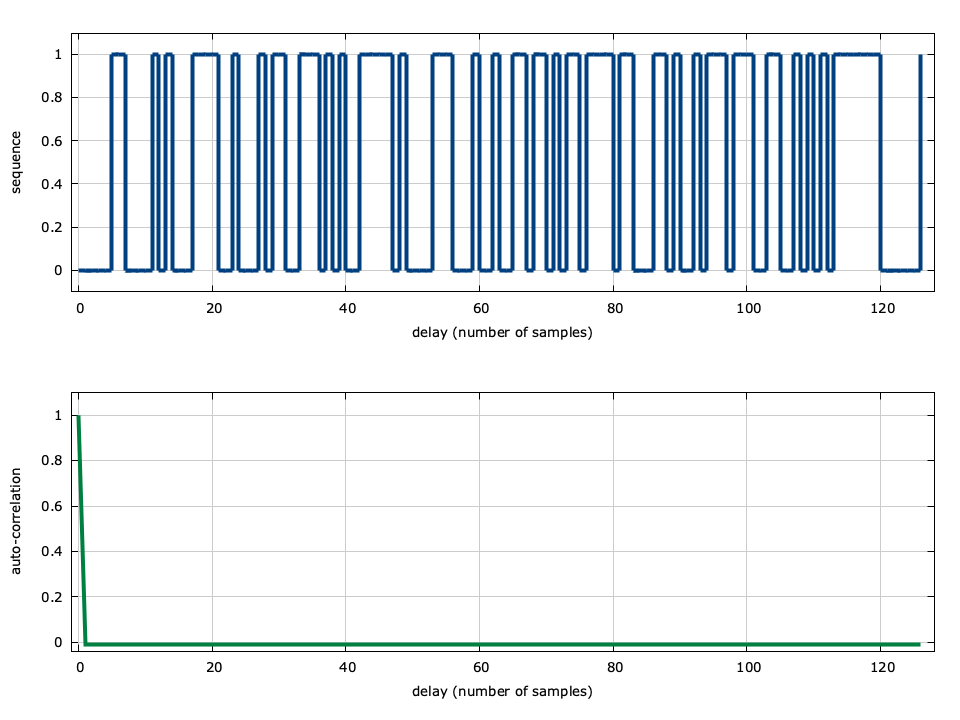# Maximal-length Sequence Generator (msequence)

The msequence object in liquid is really just a linear feedback shift register (LFSR), efficiently implemented using unsigned integers. The LFSR consists of an $$m$$ -bit shift register, $$v$$ , and generator polynomial $$g$$ . For primitive polynomials, the output sequence has a length $$n=2^m-1$$ before repeating. This sequence is known as a maximal-length P/N (positive/negative) sequence, and consists of several useful properties:

• the output sequence has very good auto-correlation properties; when aligned, the sequence, of course, correlates perfectly to 1. When misaligned by any amount, however, the sequence correlates to exactly $$-1/n$$ .
• the sequence is easily generated using a linear feedback shift register

Only a certain subset of all possible generator polynomials produce this maximal length sequence.


.. table [tab-sequence-genpoly]
caption:Default $m$-sequence generator polynomials in liquid
$m$ & $n$   & $g$ (hex) & $g$ (octal) & $g$ (binary)
2   & 3     & 0x0007  & 000007  &              111
3   & 7     & 0x000b  & 000013  &             1011
4   & 15    & 0x0013  & 000023  &            10011
5   & 31    & 0x0025  & 000045  &           100101
6   & 63    & 0x0043  & 000103  &          1000011
7   & 127   & 0x0089  & 000211  &         10001001
8   & 255   & 0x012d  & 000455  &        100101101
9   & 511   & 0x0211  & 001021  &       1000010001
10  & 1023  & 0x0409  & 002011  &      10000001001
11  & 2047  & 0x0805  & 004005  &     100000000101
12  & 4095  & 0x1053  & 010123  &    1000001010011
13  & 8191  & 0x201b  & 020033  &   10000000011011
14  & 16383 & 0x402b  & 040053  &  100000000101011
15  & 32767 & 0x8003  & 100003  & 1000000000000011


The default generator polynomials are listed in [tab-sequence-genpoly] , however many more exist.


.. footnote
A list of all $m$-sequence generator polynomials are provided in
doc/data/msequence located in the main liquid project
directory.


Notice that both the first and last bit of each generator polynomial is a 1 . This holds true for all $$m$$ -sequence generator polynomials. All generator polynomials of length $$m=2\,\,(n=3)$$ through $$m=15\,\,(n=32767)$$ are given in the data/msequence/ subdirectory of this documentation directory.

Here is a brief description of the msequence object's interface in liquid :

• msequence_create(m,g,a) creates an msequence object with an internal shift register length of $$m$$ bits using a generator polynomial $$g$$ and the initial state of the shift register $$a$$ .
• msequence_create_default(m) creates an msequence object with $$m$$ bits in the shift register using the default generator polynomial (e.g. LIQUID_MSEQUENCE_GENPOLY_M6 ). The initial state is set to 000...001 .
• msequence_destroy(ms) destroys the object ms , freeing all internal memory.
• msequence_print(ms) prints the contents of the sequence to the screen.
• msequence_advance(ms) advances the msequence object's shift register by computing the binary dot product of the register with the generator polynomial. The resulting bit is sum of 1 s modulo 2 of the dot product and is fed back into the end of the shift register, as well as returned to the user.
• msequence_generate_symbol(ms,bps) generates a pseudo-random bps -bit symbol from the shift register. This is accomplished by advancing the msequence object bps times and shifting the result back into the symbol. It is important to note that because the sequence repeats every $$n$$ bits, if the random number is an even multiple of $$n$$ , the random sequence will repeat every bps symbols. For example, if $$m=4$$ ( $$n=15$$ ) and _bps is 3, then the sequence will repeat 5 times.
• msequence_reset(ms) resets the msequence object's internal shift register to the original state (typically 000...001 ).
• msequence_get_length(ms) returns the length of the sequence, $$n$$
• msequence_get_state(ms) returns the internal state of the sequence, $$v$$Figure [fig-sequence-msequence]. msequence auto-correlation, $$m=7$$ ( $$n=127$$ ), $$g=$$ 10001001

The auto-correlation of the $$m$$ -sequence with generator polynomial $$g=$$ 1000011 can be seen in [fig-sequence-msequence] . The shift register has seven bits ( $$m=7$$ ) and therefore the output sequence is of length $$n=2^m-1=127$$ . Notice that the auto-correlation is equal to unity with no delay, and nearly zero ( $$-1/127$$ ) for all other delays.